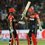# Looks the same!

$\large{ \begin{cases} a^3 = 3(b^2+c^2)-25 \\ b^3=3(c^2+a^2)-25 \\ c^3=3(a^2+b^2)-25 \end{cases}}$

Let $a,b$ and $c$ be distinct real numbers satisfying the system of equations above. Find the product $abc$.Note by Ayush G Rai
5 years, 2 months ago

This discussion board is a place to discuss our Daily Challenges and the math and science related to those challenges. Explanations are more than just a solution — they should explain the steps and thinking strategies that you used to obtain the solution. Comments should further the discussion of math and science.

When posting on Brilliant:

• Use the emojis to react to an explanation, whether you're congratulating a job well done , or just really confused .
• Ask specific questions about the challenge or the steps in somebody's explanation. Well-posed questions can add a lot to the discussion, but posting "I don't understand!" doesn't help anyone.
• Try to contribute something new to the discussion, whether it is an extension, generalization or other idea related to the challenge.

MarkdownAppears as
*italics* or _italics_ italics
**bold** or __bold__ bold
- bulleted- list
• bulleted
• list
1. numbered2. list
1. numbered
2. list
Note: you must add a full line of space before and after lists for them to show up correctly
paragraph 1paragraph 2

paragraph 1

paragraph 2

[example link](https://brilliant.org)example link
> This is a quote
This is a quote
    # I indented these lines
# 4 spaces, and now they show
# up as a code block.

print "hello world"
# I indented these lines
# 4 spaces, and now they show
# up as a code block.

print "hello world"
MathAppears as
Remember to wrap math in $$ ... $$ or $ ... $ to ensure proper formatting.
2 \times 3 $2 \times 3$
2^{34} $2^{34}$
a_{i-1} $a_{i-1}$
\frac{2}{3} $\frac{2}{3}$
\sqrt{2} $\sqrt{2}$
\sum_{i=1}^3 $\sum_{i=1}^3$
\sin \theta $\sin \theta$
\boxed{123} $\boxed{123}$

Sort by:

Suppose that we have a solution of distinct reals $(a,b,c)$. Let $S = a^2 + b^2 + c^2$.
Then, we have $a^3 + 3a^2 + 25 = 3 (a^2 + b^2 + c^2 ) = 3S$, and similar equations for $b$ and $c$.

Since these are distinct reals, we know that the roots to the cubic equation $X^3 + 3X^2 + 25 - 3S = 0$ must be $a, b, c$.
Hence, by vieta's formula, this tells us $a+b+c = -3, ab+bc+ca = 0$.

Thus, $S = (a^2+b^2+c^2) = (a+b+c)^2 - 2(ab+bc+ca) = 9$. Finally, using the constant coefficient, $abc = - ( 25 - 3S ) = - (25 - 27) = 2$.

Note: We can now solve $x^3 + 3x^2 -2 = 0$ to obtain that $(a,b,c) = ( -1, -1 - \sqrt{3}, -1 + \sqrt{3} )$.

Staff - 5 years, 2 months ago

good simple approach!

- 5 years, 2 months ago

Hint: What can we say about $a^3 + 3a^2 + 25$?

The next hint is in white text, so you will need to Toggle Latex.

Hint: For a given solution set, consider $\color{#FFFFFF} { f(x) = x^3 + 3x^2 + 25 - 3(a^2+b^2+c^2). \text{What are the roots?}}$

Staff - 5 years, 2 months ago

- 5 years, 2 months ago

Which problem? This one? If so, see the hint.

Staff - 5 years, 2 months ago

How to toggle latex??

- 5 years, 2 months ago

On browser, click on your profile iconBut otherwise, (since the above is already a spoiler space), the second hint says

For a given solution set, consider $f(x) = x^3 + 3x^2 + 25 - 3(a^2+b^2+c^2).$ What are the roots?

Staff - 5 years, 2 months ago

Sir it hence means that ABC = 2

- 5 years, 2 months ago

first subtract the equation simultaneously to get $\begin{cases} a^3-b^3=-3(a^2-b^2)\\ b^3-c^3=-3(b^2-c^2)\\ c^3-a^3=-3(c^2-a^2) \end{cases}$ since all variable are distinct we can divide both side by (a-b),(b-c),(c-a) respectively. we get $\begin{cases} a^2+ab+b^2+3(a+b)=0\\ b^2+bc+c^2+3(b+c)=0\\ c^2+ca+c^2+3(c+a)=0\\ \end{cases}$ subtract any two equation to get $a+b+c=-3$ add all the equations to get $2(a^2+b^2+c^2)+ab+bc+ca+6(a+b+c)=0$ using the fact$a^2+b^2+c^2=(a+b+c)^2-2(ab+bc+ca)=9-2(ab+bc+ca)$ $ab+bc+ca=0$ adding all the original equations $a^3+b^3+c^3=6(a^2+b^2+c^2)-75=-21$ using the identity $a^3+b^3+c^3=(a+b+c)(a^2+b^2+c^2-ab-bc-ca)+3abc=-27+3abc$ so we have $-27+3abc=-21\to abc=2$

- 5 years, 2 months ago

Awesome solution...(+1) one of the best.

- 5 years, 2 months ago

The answer is $abc=2$

- 5 years, 2 months ago

- 5 years, 2 months ago

This is from NMTC 2015 lvl 2

- 5 years, 2 months ago

you are absolutely correct.

- 5 years, 2 months ago

What is the sum of 1!+2!+3!+4!+.....+n!

- 5 years, 2 months ago

why do you want that?

- 5 years, 2 months ago

Actually, there is no neat closed form expression for $\sum_{n=1}^{k}\,n!$.

There are some formulae but you would need to know about exponential integrals and several other advance concepts for understanding them.

However, there are some series involving factorials like $\sum_{n=1}^{k}\,n \cdot n!$ which can be described by simple expressions.

- 5 years, 2 months ago